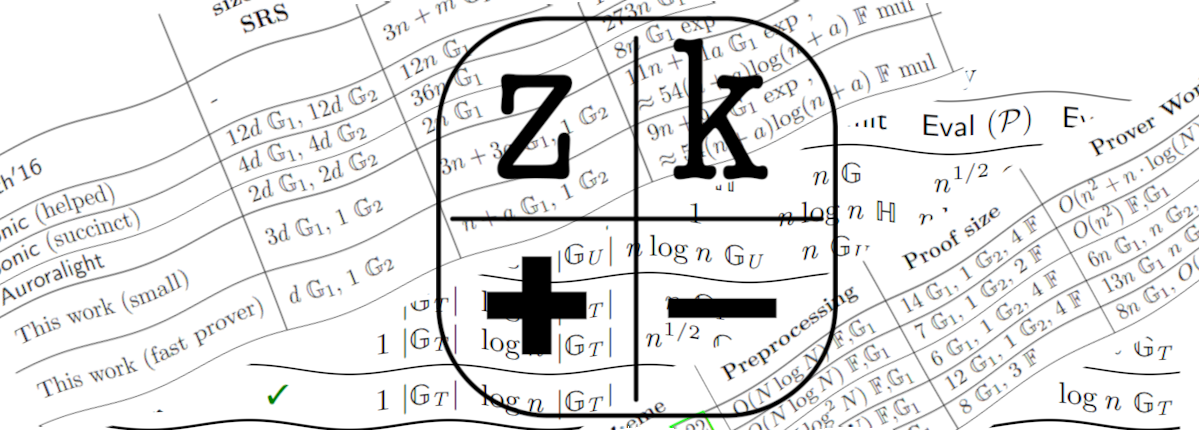• home
• blog
• research
• bounties
• team
• events

# zkalc: a cryptographic calculator

## George Kadianakis, Michele Orrù | January 16, 2023zkalc helps you calculate how much time cryptographic operations take on a real computer.

zkalc was created to instantly answer questions like "How quickly can I run an MSM of size $2^{18}$ and compute $120$ pairings on an AWS C5 machine?" or "Which curve can perform DH in less than $10$ microseconds?".

Tune in and play with it!

## Why?

Cryptographers tend to be good at cryptography but they can be quite bad at estimating the time it takes a computer to run their schemes.

We hope that zkalc can help shorten the gap between cryptography and practice:

• Cryptographers can use the simple UX to learn how fast their new fancy scheme runs on various machines without wasting computer cycles and CO2;
• Protocol designers can easily tune the parameters of their protocol depending on their requirements

We designed zkalc to be easy to use but also easy to extend. Writing new types of benchmarks, or adding fresh data to the zkalc website is easy.

## How does zkalc work?

Let's now go over our benchmarking pipeline and how we derive our results. In short:

1. For each supported operation, we run benchmarks that measure its performance. We use criterion.rs to take multiple sample (at least 10, ever for large computations like MSMs), and then select the average.
2. We collect benchmark results inside the perf/data/ directory and make them freely available for anyone to use.
3. For each operation, we fit a function to its benchmark results. We use linear interpolation inside the benchmark bounds and least squares regression outside the benchmarking bounds.
4. When a user queries zkalc for an operation of size $n$, we estimate the its running time using the produced function.

In this blog post we will go deeper into the above process. We will mainly focus on the function fitting, but if you are interested in the entire story of how our benchmarks work, or if you want to see the interactive version of the graphs below, please visit the zkalc methodology page.

### Running benchmarks

For every supported operation, we write benchmarks and run them in multiple platforms. We then store the results in the perf/ directory of zkalc.

Now we have benchmark data for every operation in the perf/ directory. The next step is to fit a function $f(x)$ to every operation, so that when a user queries us for an operation with arbitrary size $n$, we can answer it by evaluating $f(n)$.

For simple operations like basic scalar multiplication and field addition (which are not amortized) we consider them to be sequential computations. That is, if a single scalar multiplication takes $x$ seconds, $n$ such operations will take $n \cdot x$ seconds. That results in a simple linear function $f(x) = n \cdot x$.

More complicated operations like MSMs and pairing products are amortized and their performance doesn't follow a simple linear curve.

For such operations, we collect benchmark data for various sizes. For example, consider the figure below which displays the benchmark data from a $\mathbb G_1$ MSM operation for sizes from $2$ to $2^{21}$ (both axis are in log scale):To answer user queries within the benchmark range, we perform polynomial interpolation over the benchmark data.

That is, for each pair of benchmark data $(x_i, f(x_i))$ and $(x_{i+1}, f(x_{i+1}))$ we trace the line that goes through both points. We end up with a piecewise function that covers the entire benchmark range, as we can see in the figure below:For user queries outside of the benchmarking range we extrapolate via non-linear least squares. To make things more exciting we decided that it should be done... in Javascript inside your browser.

In the specific case of MSMs, Pippenger's complexity is well known to be asymptotically $O({n} / {\log n})$. Hence, we use least squares to fit the data set to a function $h(x) = \frac{a x + b}{\log x}$ solving for $a, b$.

Here is an illustration of the extrapolation behavior of $\mathbb G_1$ MSM outside of the benchmarking range (that is, after $2^{21}$):We do not expect extrapolation to faithfully follow the benchmarks. We believe however that the estimates provide a rough idea of how long an algorithm will take.

In the end of this process, we end up with a piecewise function for each operation that we can query inside and outside the benchmarking range to answer user queries.

Do give zkalc a try and let us know what you think!

## Visualizing crypto performance with zkalc

In the zkalc website, you will also find the zcharts corner where we visualize all the raw benchmark data we used in the above section.

We hope that this visual approach will help you grok the benchmark data that zkalc is based on, but also acquire a better understanding of the performance variations between different implementations/curves.

## A call for help

zkalc can be only as useful as the data it provides,and there is lots of room for additional benchmarks. Can you run benchmarks on a large cloud provider? We would love to get in touch and gather benchmarks for zkalc. Do you have access to a beefy GPU prover? We would love to help you run zkalc. Did you just design a new elliptic curve? Benchmark it with zkalc. Are you working on a new crypto library? You guessed it. Adding benchmarks to zkalc is actually not hard; check our website for instructions!

In the future, we also want to expand zkalc to support higher level primitives. From FFTs, to IPAs, to various polynomial commitment and lookup argument schemes. If you want to write benchmarks for any of these, check out our TODO file and please get in touch! :)

## Acknowledgements

Many thanks to Patrick Armino and Jonathan Xu for their help with the UX.

cryptography@ethereum.org